Share

NCERT solutions for Class 10 Mathematics chapter 2 - Polynomials

Mathematics Textbook for Class 10

NCERT Mathematics Class 10Chapter 2: Polynomials

Ex. 2.10Ex. 2.20Ex. 2.30Ex. 2.40

Chapter 2: Polynomials Exercise 2.10 solutions [Page 28]

Ex. 2.10 | Q 1.1 | Page 28

The graphs of y = p(x) are given in following figure, for some polynomials p(x). Find the number of zeroes of p(x), in the following.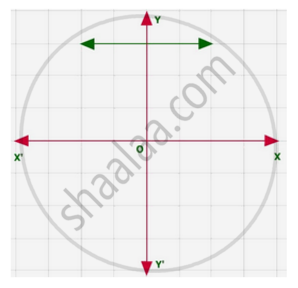Ex. 2.10 | Q 1.2 | Page 28

The graphs of y = p(x) are given in following figure, for some polynomials p(x). Find the number of zeroes of p(x)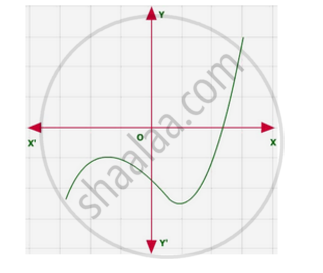Ex. 2.10 | Q 1.3 | Page 28

The graphs of y = p(x) are given in following figure, for some polynomials p(x). Find the number of zeroes of p(x)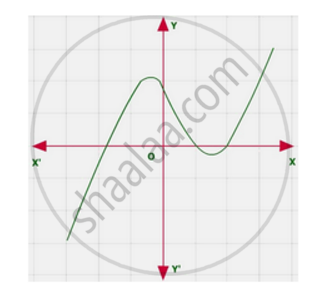Ex. 2.10 | Q 1.4 | Page 28

The graphs of y = p(x) are given in following figure, for some polynomials p(x). Find the number of zeroes of p(x)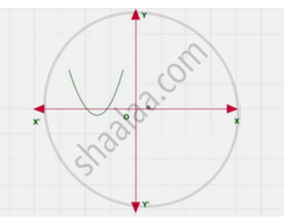Ex. 2.10 | Q 1.5 | Page 28

The graphs of y = p(x) are given in following figure, for some polynomials p(x). Find the number of zeroes of p(x)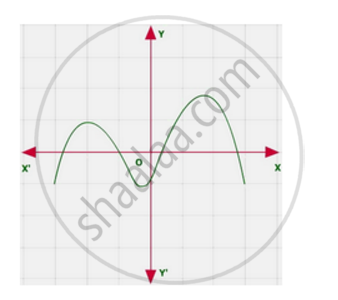Ex. 2.10 | Q 1.6 | Page 28

The graphs of y = p(x) are given in following figure, for some polynomials p(x). Find the number of zeroes of p(x)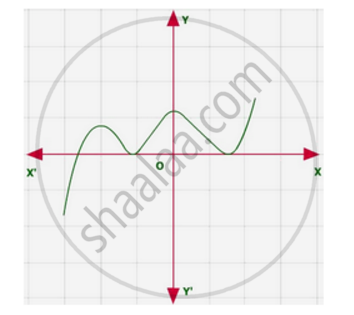Chapter 2: Polynomials Exercise 2.20 solutions [Page 33]

Ex. 2.20 | Q 1.1 | Page 33

Find the zeroes of the following quadratic polynomials and verify the relationship between the zeroes and the coefficients

x2 – 2x – 8

Ex. 2.20 | Q 1.2 | Page 33

Find the zeroes of the following quadratic polynomials and verify the relationship between the zeroes and the coefficients

4s2 – 4s + 1

Ex. 2.20 | Q 1.3 | Page 33

Find the zeroes of the following quadratic polynomials and verify the relationship between the zeroes and the coefficients.

6x2 – 3 – 7x

Ex. 2.20 | Q 1.4 | Page 33

Find the zeroes of the following quadratic polynomials and verify the relationship between the zeroes and the coefficients

4u2 + 8u

Ex. 2.20 | Q 1.5 | Page 33

Find the zeroes of the following quadratic polynomials and verify the relationship between the zeroes and the coefficients

t2 – 15

Ex. 2.20 | Q 1.6 | Page 33

Find the zeroes of the following quadratic polynomials and verify the relationship between the zeroes and the coefficients

3x2 – x – 4

Ex. 2.20 | Q 2.1 | Page 33

Find a quadratic polynomial each with the given numbers as the sum and product of its zeroes respectively

1/4 , -1

Ex. 2.20 | Q 2.2 | Page 33

Find a quadratic polynomial each with the given numbers as the sum and product of its zeroes respectively.

sqrt2 , 1/3

Ex. 2.20 | Q 2.3 | Page 33

Find a quadratic polynomial each with the given numbers as the sum and product of its zeroes respectively

0, sqrt5

Ex. 2.20 | Q 2.4 | Page 33

Find a quadratic polynomial each with the given numbers as the sum and product of its zeroes respectively

1, 1

Ex. 2.20 | Q 2.5 | Page 33

Find a quadratic polynomial each with the given numbers as the sum and product of its zeroes respectively

-1/4 ,1/4

Ex. 2.20 | Q 2.6 | Page 33

Find a quadratic polynomial each with the given numbers as the sum and product of its zeroes respectively

4, 1

Chapter 2: Polynomials Exercise 2.30 solutions [Page 36]

Ex. 2.30 | Q 1.1 | Page 36

Divide the polynomial p(x) by the polynomial g(x) and find the quotient and remainder in each of the following

p(x) = x3 – 3x2 + 5x – 3, g(x) = x2 – 2

Ex. 2.30 | Q 1.2 | Page 36

Divide the polynomial p(x) by the polynomial g(x) and find the quotient and remainder in each of the following : p(x) = x4 – 3x2 + 4x + 5,   g(x) = x2 + 1 – x

Ex. 2.30 | Q 1.3 | Page 36

Divide the polynomial p(x) by the polynomial g(x) and find the quotient and remainder in each of the following

p(x) = x4 – 5x + 6, g(x) = 2 – x2

Ex. 2.30 | Q 2.1 | Page 36

Check whether the first polynomial is a factor of the second polynomial by dividing the second polynomial by the first polynomial

t2 – 3, 2t4 + 3t3 – 2t2 – 9t – 12

Ex. 2.30 | Q 2.2 | Page 36

Check whether the first polynomial is a factor of the second polynomial by dividing the second polynomial by the first polynomial

x2 + 3x + 1, 3x4 + 5x3 – 7x2 + 2x + 2

Ex. 2.30 | Q 2.3 | Page 36

Check whether the first polynomial is a factor of the second polynomial by dividing the second polynomial by the first polynomial

x3 – 3x + 1, x5 – 4x3 + x2 + 3x + 1

Ex. 2.30 | Q 3 | Page 36

Obtain all other zeroes of 3x4 + 6x3 – 2x2 – 10x – 5, if two of its zeroes are sqrt(5/3) and - sqrt(5/3)

Ex. 2.30 | Q 4 | Page 36

On dividing x3 – 3x2 + x + 2 by a polynomial g(x), the quotient and remainder were x – 2 and –2x + 4, respectively. Find g(x)

Ex. 2.30 | Q 5.1 | Page 36

Give examples of polynomials p(x), g(x), q(x) and r(x), which satisfy the division algorithm

deg p(x) = deg q(x)

Ex. 2.30 | Q 5.2 | Page 36

Give examples of polynomials p(x), g(x), q(x) and r(x), which satisfy the division algorithm

deg q(x) = deg r(x)

Ex. 2.30 | Q 5.3 | Page 36

Give examples of polynomials p(x), g(x), q(x) and r(x), which satisfy the division algorithm

deg r(x) = 0

Chapter 2: Polynomials Exercise 2.40 solutions [Pages 36 - 37]

Ex. 2.40 | Q 1.1 | Page 36

Verify that the numbers given along side of the cubic polynomials are their zeroes. Also verify the relationship between the zeroes and the coefficients.

2x^3 + x^2 – 5x + 2 ; 1/2, 1, – 2

Ex. 2.40 | Q 1.2 | Page 36

Verify that the numbers given alongside of the cubic polynomials below are their zeroes. Also verify the relationship between the zeroes and the coefficients in each case

x3 – 4x2 + 5x – 2; 2, 1, 1

Ex. 2.40 | Q 2 | Page 36

Find a cubic polynomial with the sum, sum of the product of its zeroes taken two at a time, and the product of its zeroes as 2, − 7, − 14 respectively

Ex. 2.40 | Q 3 | Page 37

If the zeroes of the polynomial x3 – 3x2 + x + 1 are a – b, a, a + b, find a and b

Ex. 2.40 | Q 4 | Page 37

If two zeroes of the polynomial x4 – 6x3 – 26x2 + 138x – 35 are 2 ± sqrt3 , find other zeroes

Ex. 2.40 | Q 5 | Page 37

If the polynomial x4 – 6x3 + 16x2 – 25x + 10 is divided by another polynomial x2 – 2x + k, the remainder comes out to be x + a, find k and a.

Chapter 2: Polynomials

Ex. 2.10Ex. 2.20Ex. 2.30Ex. 2.40

NCERT Mathematics Class 10NCERT solutions for Class 10 Mathematics chapter 2 - Polynomials

NCERT solutions for Class 10 Maths chapter 2 (Polynomials) include all questions with solution and detail explanation. This will clear students doubts about any question and improve application skills while preparing for board exams. The detailed, step-by-step solutions will help you understand the concepts better and clear your confusions, if any. Shaalaa.com has the CBSE Mathematics Textbook for Class 10 solutions in a manner that help students grasp basic concepts better and faster.

Further, we at Shaalaa.com are providing such solutions so that students can prepare for written exams. NCERT textbook solutions can be a core help for self-study and acts as a perfect self-help guidance for students.

Concepts covered in Class 10 Mathematics chapter 2 Polynomials are Introduction to Polynomials, Geometrical Meaning of the Zeroes of a Polynomial, Relationship Between Zeroes and Coefficients of a Polynomial, Division Algorithm for Polynomials, Polynomials Examples and Solutions.

Using NCERT Class 10 solutions Polynomials exercise by students are an easy way to prepare for the exams, as they involve solutions arranged chapter-wise also page wise. The questions involved in NCERT Solutions are important questions that can be asked in the final exam. Maximum students of CBSE Class 10 prefer NCERT Textbook Solutions to score more in exam.

Get the free view of chapter 2 Polynomials Class 10 extra questions for Maths and can use Shaalaa.com to keep it handy for your exam preparation

S